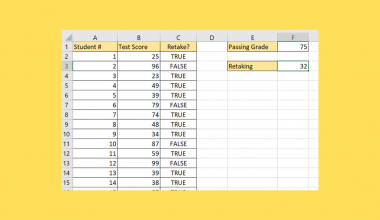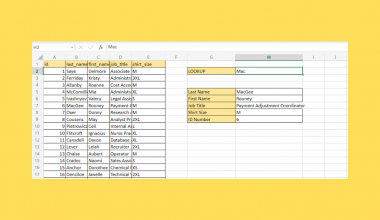# How to Find the Position of the Nth Space on a String in Excel

The FIND function in Excel is useful when you need to find the position of the nth space on a given string.

Users can use FIND to locate a substring within a larger text string. This same method can be used to find the nth occurrence of any arbitrary character.

The rules for using the `FIND` function in Excel are as follows:

• The function requires two arguments: the string to look for and the text we’ll search.
• The function then outputs the starting position of the first found instance within the given text string.
• You can also add an optional argument to specify a different position to start your search.

Let’s take a look at a quick example of a situation where we can use the `FIND` function to look for a particular instance of a character.

Suppose we have a collection of numbers separated by a space. For example, the string “4 8 15 16 23 42” has six numbers separated by spaces.

We can use a function like `MID` to return a substring of this number. But before we get the substring, we must first know the positions the substring begins and ends.

To get the second number, we must first know two values: (a) the position of the first space and (b) the position of the second space. The substring containing the second number must start one place after (a) and must end one space before (b).

We can use the `FIND` function to get the first and second occurrences of the space character in a given string. This use case is just one way where finding the position of the nth occurrence of a character can be helpful.

Now that we know when we can use the `FIND` function let’s dive into how we can use it on an actual sample spreadsheet.

## A Real Example of Finding the Position of Nth Space on String in Excel

Let’s take a look at a real example of the `FIND` function being used in an Excel spreadsheet to find an occurrence of the space character.

In the example below, we’ve used the `FIND` function to find the first instance of a space character in the provided text. Because of the formula, we now know that the space is the fifth character in the string.To get the values in cell B13, we just need to use the following formula:

`=FIND(“ “,A13)`

The first argument is the substring we want to search for. In this case, we simply want to look for a single space.

In this second example, we are looking for a hyphen instead. We’re also looking for the second instance of the hyphen rather than just the first. Since the `FIND` function only returns the first instance, we’ll have to specify a different position to start our position.The formula to get the value in cell B17 is as follows:

`=FIND("-",A17,FIND("-",A17)+1)`

To find the second hyphen, we would first have to find the position of the first. Once we get this position, we can increment the result by 1 to get the new position to start our substring search.

If you’re ready to try out the `FIND` function in Excel, try writing it yourself using the step-by-step guide in the next section!

## How to Find the Position of the Nth Space on String in Excel

This section will guide you through each step needed to start using the `FIND` function in Excel. You’ll learn how we can use this function to get the first occurrence of the space character in a string.

Follow these steps to start using the `FIND` function:

1. First, select the cell where we will place our `FIND` formula. In this example, we’ll place our first formula in cell B2.2. Next, we type in our `FIND` formula with the necessary arguments. In this example, we’ll be finding the space character in the text found in cell A2.3. Hit the Enter key to return the position of the first occurrence.4. We can drag down the formula in cell B2 to fill up the rest of the column.5. We can continue to find the next few occurrences of the space character by tweaking the formula. To get the first, and second occurrence, we can use the formula `=FIND(" ";A2;B2+1)`.
Since we already got the position of the first occurrence, we can increment it by one to specify a new starting position to find the substring.1. Why does my formula return a #VALUE! error?
If the `FIND` function returns a #VALUE! error, this tells you that the substring could not be found in the provided text. If you want to catch this error, you can use the `IFERROR` function to return something else when the substring is not found.

That’s all you need to remember to start using the `FIND` function in Excel to find the position of the nth space in a given string. This step-by-step guide should be enough for you to find substrings in cells with text accurately.

The `FIND` function is just one example of a text function you can use in Microsoft Excel. With so many other Excel functions out there, there are surely a few that can help improve your spreadsheets.

Are you interested in learning more about what Excel can do? Stay notified of new guides like this by subscribing to our newsletter!### Get emails from us about Excel.

Our goal this year is to create lots of rich, bite-sized tutorials for Excel users like you. If you liked this one, you'd love what we are working on! Readers receive ✨ early access ✨ to new content.

##### You May Also Like## How to Use Less Than or Equal to Operator in Excel

The less than or equal to operator in Excel is useful when you need to know if a…## How to Create a Population Pyramid Chart in Excel

A population pyramid chart is useful when you indeed to illustrate the distribution of a population by age…## How to Insert Live Exchange Rate in Excel

We can use the Currencies data type in Excel to insert live exchange rate data in Excel. The…## How to Extract a String from an IP Address in Excel

This guide will show how easy it is to extract a particular string from any IP address using…